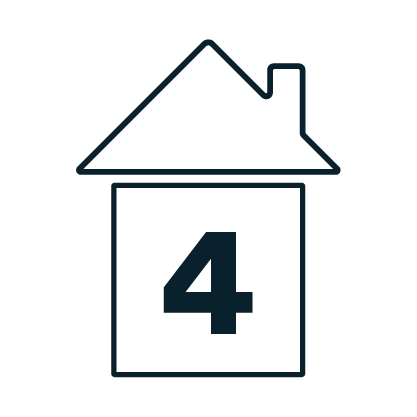##Grade 4 Module 5 Lesson 21

How can we use tape diagrams, number lines, and number bonds to add fractions? Join Ms. DelFavero for this lesson where we’ll use mathematical models to add fractions with related units. For this lesson, you’ll need paper and pencil. If you have access to a workbook, or a printer, we also suggest using the Problem Set available using the link below the video